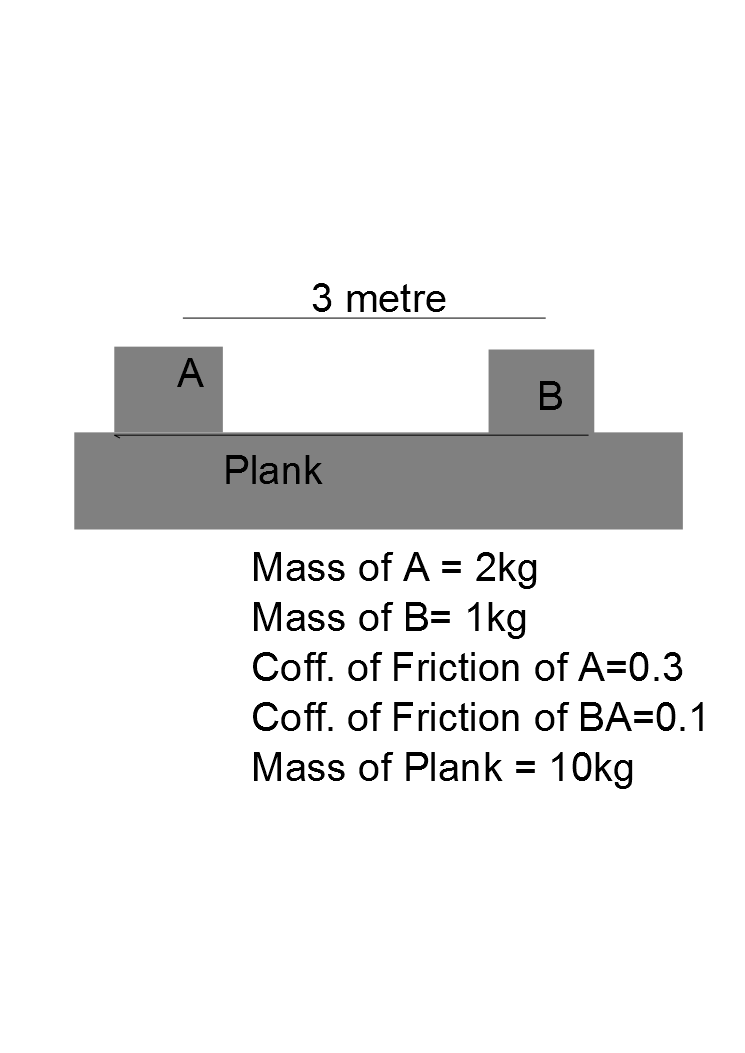What You Will Take As system.A plank of mass 10kg rests on a smooth horizontal surface. Two blocks A and B of masses 2kg and 1 kg respectively. Distance b/w both masses is 3 metre. Coff. of friction for A is 0.3 and for B is 0.1 . Now a force of 15 N is applied to the plank in Horizontal Direction. Find the time after which Block A collides with B.

Round Off to nearest Integer.

Note : Correct the 2nd last statement as coff. of friction for B.

×